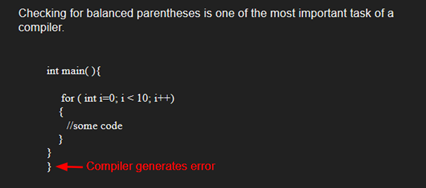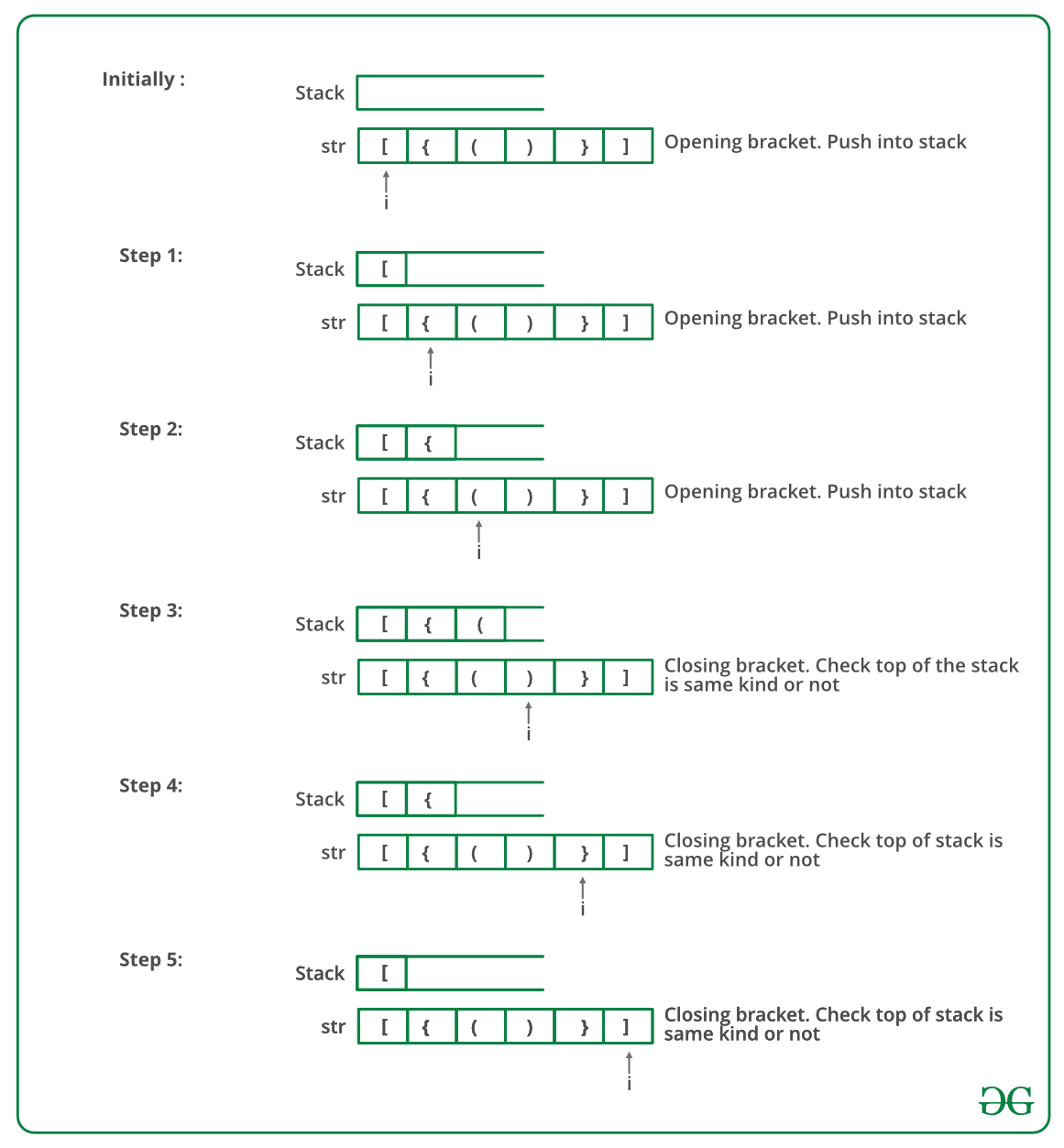Open in App
Not now

# C++ Program To Check For Balanced Brackets In An Expression (Well-Formedness) Using Stack

• Difficulty Level : Medium
• Last Updated : 14 Dec, 2021

Given an expression string exp, write a program to examine whether the pairs and the orders of “{“, “}”, “(“, “)”, “[“, “]” are correct in exp.

Example

Input: exp = “[()]{}{[()()]()}”
Output: Balanced

Input: exp = “[(])”
Output: Not BalancedAlgorithm:

• Declare a character stack S.
• Now traverse the expression string exp.
1. If the current character is a starting bracket (‘(‘ or ‘{‘ or ‘[‘) then push it to stack.
2. If the current character is a closing bracket (‘)’ or ‘}’ or ‘]’) then pop from stack and if the popped character is the matching starting bracket then fine else brackets are not balanced.
• After complete traversal, if there is some starting bracket left in stack then “not balanced”

Below image is a dry run of the above approach:Below is the implementation of the above approach:

## C++

 `// CPP program to check for balanced brackets. ` `#include ` `using` `namespace` `std; ` ` `  `// function to check if brackets are balanced ` `bool` `areBracketsBalanced(string expr) ` `{   ` `    ``stack<``char``> s; ` `    ``char` `x; ` ` `  `    ``// Traversing the Expression ` `    ``for` `(``int` `i = 0; i < expr.length(); i++)  ` `    ``{ ` `        ``if` `(expr[i] == ``'('` `|| expr[i] == ``'['` `            ``|| expr[i] == ``'{'``)  ` `        ``{ ` `            ``// Push the element in the stack ` `            ``s.push(expr[i]); ` `            ``continue``; ` `        ``} ` ` `  `        ``// IF current current character is not opening ` `        ``// bracket, then it must be closing. So stack ` `        ``// cannot be empty at this point. ` `        ``if` `(s.empty()) ` `            ``return` `false``; ` ` `  `        ``switch` `(expr[i]) { ` `        ``case` `')'``: ` `             `  `            ``// Store the top element in a ` `            ``x = s.top(); ` `            ``s.pop(); ` `            ``if` `(x == ``'{'` `|| x == ``'['``) ` `                ``return` `false``; ` `            ``break``; ` ` `  `        ``case` `'}'``: ` ` `  `            ``// Store the top element in b ` `            ``x = s.top(); ` `            ``s.pop(); ` `            ``if` `(x == ``'('` `|| x == ``'['``) ` `                ``return` `false``; ` `            ``break``; ` ` `  `        ``case` `']'``: ` ` `  `            ``// Store the top element in c ` `            ``x = s.top(); ` `            ``s.pop(); ` `            ``if` `(x == ``'('` `|| x == ``'{'``) ` `                ``return` `false``; ` `            ``break``; ` `        ``} ` `    ``} ` ` `  `    ``// Check Empty Stack ` `    ``return` `(s.empty()); ` `} ` ` `  `// Driver code ` `int` `main() ` `{ ` `    ``string expr = ``"{()}[]"``; ` ` `  `    ``// Function call ` `    ``if` `(areBracketsBalanced(expr)) ` `        ``cout << ``"Balanced"``; ` `    ``else` `        ``cout << ``"Not Balanced"``; ` `    ``return` `0; ` `} `

Output

`Balanced`

Time Complexity: O(n)
Auxiliary Space: O(n) for stack.

Please refer complete article on Check for Balanced Brackets in an expression (well-formedness) using Stack for more details!

My Personal Notes arrow_drop_up
Related Articles